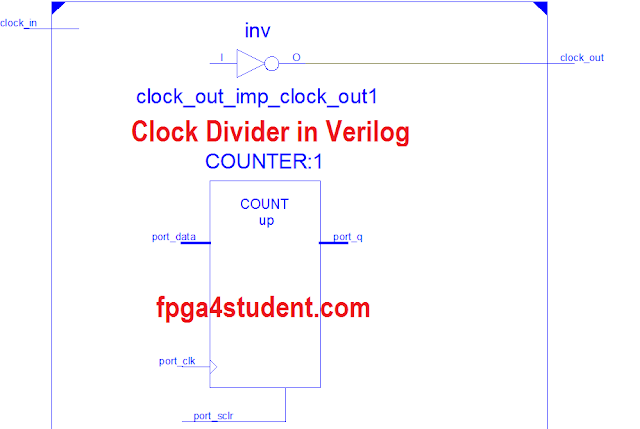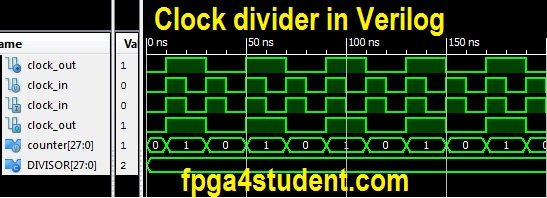# Verilog code for Clock divider on FPGA

## Last time, I presented a VHDL code for a clock divider on FPGA. This Verilog project provides full Verilog code for the Clock Divider on FPGA together with Testbench for simulation. The Verilog clock divider is simulated and verified on FPGA.The frequency of the output clock_out is equal to the frequency of the input clock_out divided by the value of the DIVISOR parameter in the Verilog code.
F(clock_out) = F(clock_in)/DIVISOR
To change the clock frequency of the clock_out, just modify the DIVISOR parameter.

### Verilog code for the clock divider on FPGA:

```// fpga4student.com: FPGA projects, VHDL projects, Verilog projects
// Verilog project: Verilog code for clock divider on FPGA
// Top level Verilog code for clock divider on FPGA
module Clock_divider(clock_in,clock_out
);
input clock_in; // input clock on FPGA
output reg clock_out; // output clock after dividing the input clock by divisor
reg[27:0] counter=28'd0;
parameter DIVISOR = 28'd2;
// The frequency of the output clk_out
//  = The frequency of the input clk_in divided by DIVISOR
// For example: Fclk_in = 50Mhz, if you want to get 1Hz signal to blink LEDs
// You will modify the DIVISOR parameter value to 28'd50.000.000
// Then the frequency of the output clk_out = 50Mhz/50.000.000 = 1Hz
always @(posedge clock_in)
begin
counter <= counter + 28'd1;
if(counter>=(DIVISOR-1))
counter <= 28'd0;```
``` clock_out <= (counter<DIVISOR/2)?1'b1:1'b0;end
endmodule```

### Verilog Testbench code for the clock divider on FPGA:

````timescale 1ns / 1ps
// fpga4student.com FPGA projects, VHDL projects, Verilog projects
// Verilog project: Verilog code for clock divider on FPGA
// Testbench Verilog code for clock divider on FPGA
module tb_clock_divider;
// Inputs
reg clock_in;
// Outputs
wire clock_out;
// Instantiate the Unit Under Test (UUT)
// Test the clock divider in Verilog
Clock_divider uut (
.clock_in(clock_in),
.clock_out(clock_out)
);
initial begin
// Initialize Inputs
clock_in = 0;
// create input clock 50MHz
forever #10 clock_in = ~clock_in;
end

endmodule```

#### Simulation waveform for the clock divider in Verilog:It is noted that this code is about to create another clock in your design, so the FPGA tools required to take care of an extra internally generated clock during clock tree synthesis, which might cause FPGA timing issues as it is not generated by dedicated FPGA clock generators (PLL/DCM/etc). In addition, you also have to take care of the multi-clock domain issues while designing such as interfacing signals between different clock domains (synchronizer needed, etc). It is recommended on FPGA to generate a slower clock enable signal instead to drive another part of your design. You can visit here for more details on how to do it in Verilog.
Recommended Verilog projects:

1.Isn't there going to be inferred latches in the example code?

1.No, the counter will be synthesized as registers (FFs).

2.So if we wish to modify the duty cycle, we change the dividing value in the assign state right? divisor/2 = 50% dc, divisor/4 =25% dc and so on.. correct?

1.Yes, duty cycle can be modified by that. Note that duty cycle won't not be 50% with the odd DIVISORs.

3.Will this divide clock by 2,4,6,8 and so on?

1.Sure, just change the DIVISOR.

4.Hello, If I want to change the Frecuency how do I modify the Divider? I have to do it in the assign or the parameter?